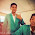## Saturday, March 6, 2010

### How to round numbers

There is a versatile function in Ax to perform rounding operations: round.
This function rounds the first real argument to the nearest multiple of the second real argument. So plenty of possibilities, for example

round ( 1.2 , 1) equals 1
round ( 1.2 , 5) equals 0
round ( 6.4 , 5) equals 5
round ( 7.5 , 5) equals 10
round ( 1.2 , 0.5) equals 1
round ( 1.12 , 0.1) equals 1.1

If you don't want to work with the multiples of the second argument and instead just want to specify a number of decimals places to round, you can use decround.
This functions rounds the first real argument to the number of decimals specified (second argument). So for example

decround (1.2 , 0) equals 1
decround (1.23 , 0) equals 1
decround (1.23 , 1) equals 1.2
decround (1.25 , 1) equals 1.3

But the second argument can be negative as well. Like this:

decround (123, -2) equals 100

Now for rounding with a little twist: roundup.

If you want to round a number up to the next real value, you can use roundup. This function is the same as the ceiling function from other environments.
Same format as the previous round functions, needing 2 arguments.
So for example

roundup ( 1.23 , 0.1) give as result 1.3
roundup ( 123 , 5) equals 125
roundup ( 123.23 , 5) equals 125
roundup ( 123.23 , 1) equals 124

If that ain't enough, more rounding functions: rounddown and roundzero.
Rounddown rounds your real value always down to the multiple of your second argument.
While roundzero, as the function name says, rounds towards zero.
The difference you can see in the next example:

rounddown (-9 , 5) equals -10
roundzero (-9 , 5) equals -5

1.This is a great post!

2.3.This comment has been removed by the author.

4.5.how bout rounding with more than 2 decimal places?

6.how bout rounding with more than 2 decimal places?

1.static void round_decimal(Args _args)
{
real temp, roundingUp, roundingDown;
;
temp = 61.7032;
roundingUp = roundUp(temp, 1);
roundingDown = roundDown(temp, 1);
info(strFmt("Origin : %1 RoundingUp : %2 RoundingDown : %3", temp, RoundingUp, roundingDown));
}

7.This is awesome. Thanks for this!